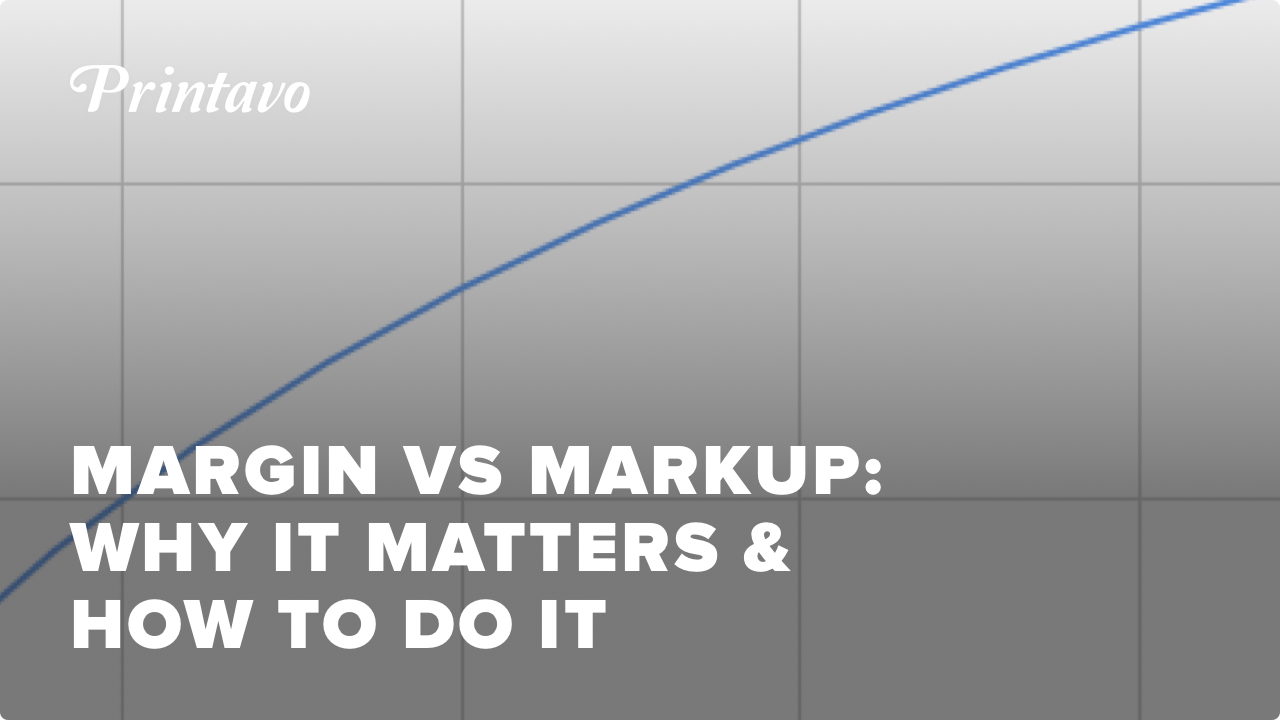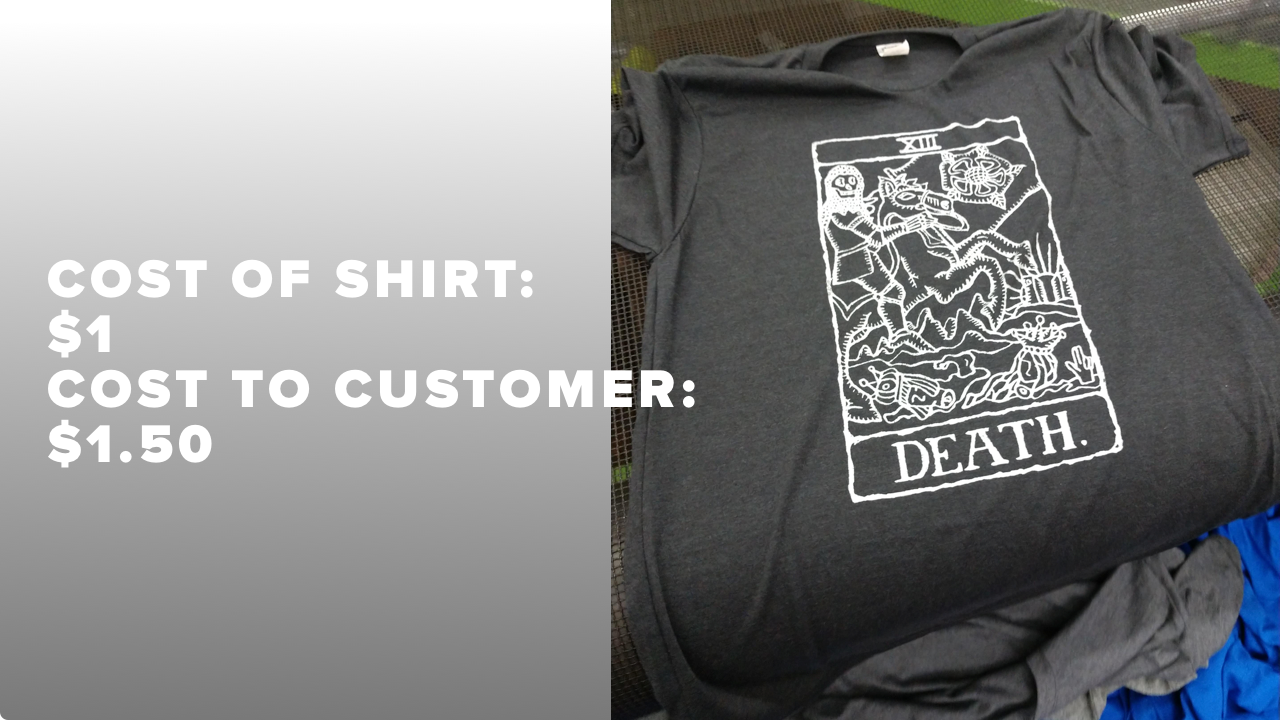# Markup vs. Margin | Not The Same! | How To Calculate and Price Your Margins & MarkupsScheduling, quoting, approvals, payments, customer communication, automation and more. With Printavo, you’ll work smarter–not harder.

Small business owners confuse markup and margin all the time.

We want to clear things up for everyone!

So let's dig in and show you the difference between your markup and your margin.

## Markup & Margin Terminology

Understanding margins and markup are crucial to success in any business. But in screen printing, understanding the relationship between your gross margins, your markup percentages, and your gross profits can be challenging. "It seems obvious, but every job in screen printing is different," explained Golden Press Studio.

Let's cover the basic terminology involved with markups and margin calculations.

### Cost of Goods Sold (COGS)

Traditionally, cost of goods sold is simply the price that you pay for whatever items you sell.

COGS seems simple: how much did it cost you to sell your product?

It's ultimately a simplified view of how expensive it is for your company to produce a t-shirt.

COGS includes labor, the cost of the t-shirt, inks, time spent administering and selling the job, and so on.

You most likely have an intuitive (though not always accurate) sense of your COGS. Think of it this way: it's what you subtract from your selling price to determine your profit!

### Revenue / Sell Price

This is, quite simply, the amount you can sell a product for.

If you sell a shirt for \$20, that's your revenue.

Note: when calculating a margin, most screen printers take the margin of the entire order into account – not the margin on one t-shirt.

### Gross Profits

You'll also need to estimate (or know) your gross profits on any order.

Realistically, shops break into two major camps around profits:

• Group 1 (Majority) – Profits are whatever is left over after each transaction (or the end of the month, etc.).
• Group 2 (Minority) – Profits are a carefully planned percentage of every transaction.

Regardless, your gross profits are the dollar amount that you take in as profit after all of your overhead and costs are paid for. Think of your gross profits as your ultimate bottom line.

Profits are, simply, why you're in business!

## What are "margins" / profit margins?

Margins are how you understand the health and sustainability of your business.

They're a big-picture view of how much profit you can expect to make.

Margins involve your pricing, so they're sensitive to each order you take and how much overhead it incurs.

### How to calculate margins / profit margins

To calculate your margin, we use a simple formula:

(Gross profits / Revenue) * 100 = Margin %

## What is markup?

Markups are thrown around a lot in conversations incorrectly as a shorthand for profit margins.

You'll often hear someone refer to "markup" as the amount of the selling price that's above the cost of the goods – implying that all of that amount is profit.

That's partially true. Markup is how much money your customer is pays above the wholesale price of a blank garment.

But markups are only a small-picture view of how much you're increasing the price of each garment by. It doesn't include revenue, which is how your actual margins are determined.

### How to calculate markup

To calculate your markup, we use a very simple formula:

(Gross profits / Cost of goods) * 100 = Markup %

## What's the difference between markup and margin?Let's give you a concrete (but very simplified) example to demonstrate markup versus margin.

You purchase a t-shirt from a vendor at \$1.00 (your COGS).

On this sale, we assume your gross profits are \$0.50.

### Markup

This sale, clearly, has a 50% markup.

Plugging in our specific example, this is what we get when we use our markup equation:

(Gross profits (\$0.50) / Cost of goods (\$1.00)) * 100 = 50% markup

### Margin

However, this sale has a 33% margin.

Plugging in the values from our example, this is what we get when we use our margin equation:

(Gross profits (\$0.50) / Revenue (\$1.50)) * 100 = 33.3% margin

## Why margin vs. markup matters

What does all of this math this mean, specifically?

Your markup is NOT the same as your margin.

In this model, your margins will be equal to or lower than your markups.

With a firm grasp of these basic concepts, you can chart a course toward profitability, adjust your prices to get there, and have a better vision for where you are in relation to your revenue and profitability goals.

### Margins are predictable

Predictability is key!

Margin is used to calculate your future financials.

Predictable profits are the only route to long-term sustainability. If you don't know your margin and how it relates to your markup, you may miscalculate your future financials.

### Markups are how you control pricing

Pricing relates to markup, which relates to margins.

Markup is the simplest way to manipulate your pricing. If you charge slightly more per unit, your margins increase slightly.

Raising your markup by a certain percentage does not raise your margin by an equivalent percentage.

This is the key insight – and point of confusion – for many business owners.

## How to convert markup to margin

If you want to convert a specific markup to a specific margin, here's how to do it.

First, calculate your markup as a dollar value.

Second, divide the markup dollar value by the selling price.

### An example

Here's how we would do it for a shirt that has a COGS of \$1.00, a sell price of \$1.50, and therefore a markup of 50%.

First: our markup as a dollar value is \$0.50.

Second: divide our markup (\$0.50) by the sell price (\$1.50). This is equal to 33% – which is our margin.

## How to convert margin to markup

Now, let's go the other way!

If you want to convert a specific gross margin to a specific markup, here's how to do it.

First, multiply the sale price by the gross margin. This will give the gross margin in dollars.

Second, subtract the gross margin in dollars from the sell price. This gives you the COGS of the item.

Third, divide the gross margin in dollars by the COGS, then multiply by 100, to give you the markup percentage.

### An example

Here's how we would do it for a shirt that has a sell price of \$1.50 and a gross margin of 33%.

First: 33% of \$1.50 is equal to \$0.50 – the gross margin in dollars.

Second: subtract \$0.50 (the gross margin in dollars) from \$1.50 (the sell price). That's \$1 – the COGS.

Third: divide \$0.50 (the gross margin in dollars) by \$1 (the COGS) to get 0.5.

Multiply this by 100 to convert it to our markup as a percentage: 50%.

## Calculating prices from a desired margin

So, let's say you want to achieve a specific percentage margin on a job you're printing. This is quite an easy calculation to make, and you only need a couple of pieces of information:

• The cost of the goods sold.
• It's recommended that you include production costs in this number.

### How to do it

Let's go back to our simplified example: a \$1 shirt sold for \$1.50.

The margin is only 33.3%. but we actually want to achieve a 50% margin.

How would you calculate the amount to raise your price by?Here's how this is done:

Target price = Cost of goods / 1 - Desired margin

Since we know the cost of goods (\$1) and our desired margin (50%), we can easily calculate our new price. Note: we must convert our percentage (50%) to a decimal (0.5) for this formula to work.

Target price (\$x) = Cost of goods (\$1) / 1 - Desired margin (0.5)

Target price for a 50% margin = \$2

This gives us a new gross profit of \$1 and a new revenue of \$2.

### Checking our work – is this right?

Now, let's use our earlier formulas to ensure this calculation achieves what we want it to. First, we'll check that the margin is correct:

(New gross profit (\$1) / New revenue (\$2)) * 100 = 50% margin

Then we'll quickly check to see what our new markup percentage is:

(New gross profit (\$1) / Cost of goods (\$1)) * 100 = 100% markup

In other words, our markup doubled (from 50% to 100%) while our margin only increased by 66% (from 33% to 50%).

## Calculating prices from a desired markup

Calculating your price from a desired markup is very easy.

Simply multiply the cost of goods (COGS) by the desired markup percentage and add the two number together.

If we wanted to know what price to charge for a 150% markup on our \$1 t-shirt, we would multiply \$1 by 1.5. That equals \$1.50. Then we would add \$1 and \$1.50 together.

That makes our sale price \$2.50 for a 150% markup.

## Conclusion

Don't want to use a calculation?